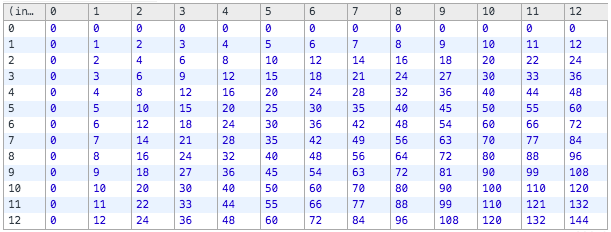# nummy

2.1.1 • Public • Published

# Nummy

Nummy is a functional and modular number computation, description, and formatting library.

## Usage

### Single-method

### A note about method signatures

You have probably noticed that different method signatures have been used above. Nummy will fill in the first argument to any method with the number it was initialized with when the method is called with fewer arguments than its full complement of arguments. This allows for greater flexibility because you can Nummy-ize a number and then call several of Nummy's methods on it:

You can also initialize an empty Nummy instance and perform different operations on different numbers:

## API Docs ### String methods

#### Base conversion

All of the base conversion methods follow the same signature: method(number**, padTo)

• #base36: `nummy(135).base36(4) => "003R"`
• #binary: `nummy(9740).binary(16) => "0010011000001100"`
• #hex: `nummy(7178).hex(8) => "00001C0A"`

#### Format numbers according to common unit systems

• #abbr: `nummy(7645).abbr(3) => "7.645k"`
• #bytes: `nummy(1478).bytes(2) => "1.44kB"`
• #metric: `nummy(6918).metric(1) => "6.9k"`

All three methods take the same first two arguments, (num**, precision)--`num` being the number to be formatted and `precision` the number of decimal places to include.

Both `bytes` and `metric` also take a `limit` argument which indicates the highest unit symbol to represent in 1000s (or 1024s for `bytes`) blocks. For `metric`, the default is 1 meaning we stop incrementing units at 1000, which works quite well for representing grams and meters (NOTE: the 'c' or hundredths unit is skipped). For `bytes`, the default is 4 meaning we stop at the trillions or 'T'. The limit can be increased or set to false to allow display of higher unit symbols.

• #chr: `nummy(77).chr() => "M"`
• #format: nummy(8822).format([optional: {place: null, thousands: ',', decimal: '.'}]) => "8,822"
• #ordinalize: nummy(1257).ordinalize() => "1257th"
### Mathematical operation methods:

#### Single argument methods

The following methods all have a single argument signature: method(number**)

• #abs: `nummy(-8635).abs() => 1`
• #acos: `nummy(0).acos() => 1.5707963267948966`
• #asin: `nummy(-0.6).asin() => -0.6435011087932844`
• #atan: `nummy(2).atan() => 1.1071487177940906`
• #ceil: `nummy(8688.5).ceil() => 8689`
• #cos: `nummy(1494).cos() => 0.17183612697016132`
• #exp: `nummy(1).exp() => 2.718281828459045`
• #floor: `nummy(748.9).floor() => 748`
• #sin: `nummy(1).sin() => 0.8414709848078965`
• #sqrt: `nummy(3950).sqrt() => 62.849025449882674`
• #tan: `nummy(1.1).tan() => 1.9647596572486523`

#### Two argument methods

The following methods all have a two argument signature: method(number1**, number2)

• #add: (alias: plus) `nummy(3137).add(1) => 3138`
• #atan2: `nummy(3641).atan2(0) => 1.5707963267948966`
• #divide: `nummy(7226).divide(2) => 0.0002767783005812344`
• #divideBy: `nummy(7226).divideBy(2) => 3613`
• #log: `nummy(3807).log() => 8.244596756382498` or `nummy(3807).log(0.2) => -5.122655986097307`
• #modulo: (alias: mod) `nummy(4508).modulo(3) => 2`
• #moduloOf: `nummy(3).moduloOf(4508) => 2`
• #multiply: `nummy(7435).multiply(2) => 14870`
• #pow: `nummy(3).pow(3) => 27`
• #round: `nummy(65).round(-1) => 70`
• #subtract: (alias: minus) `nummy(5283).subtract(1) => 5282`
• #subtractFrom: `nummy(7908).subtractFrom(0) => -7908`

#### Multi-argument methods

The following methods all have a multiple argument signature: method([num1**[, num2, …]])

• #max: returns the largest number amongst its arguments `nummy(1347).max(1, 1253, 932) => 1347`
• #min: returns the smallest number amongst its arguments `nummy(8105).min(9234, 8102) => 8102`
• #product: returns the result of multiplying all of its arguments together `nummy(1).product(2,3,4) => 24`
• #sum: returns the result of adding all of its arguments together `nummy(1).sum(2,3,4) => 10`
### Casting and Conversion methods
• #toInteger: `nummy(-735.2685004007071).toInteger() => -735`
• #toInt32: `nummy(759630119195208).toInt32() => 728388168`
• #toUInt32: `nummy(-1).toUInt32() => 4294967295`
• #toNumber: `nummy('-482.50145046040416').toNumber() => -482.50145046040416`
### Test (is) methods

#### Single argument methods

The following methods all have a single argument signature: method(number**)

• #isEven: `nummy(5629).isEven() => false`
• #isInteger: `nummy(1433).isInteger() => true`
• #isOdd: `nummy(6296).isOdd() => false`

#### Two argument methods

The following methods all have a two argument signature: method(number1**, number2)

• #isMultipleOf: `nummy(832).isMultipleOf(3) => false`
• #isFactorOf: `nummy(3).isFactorOf(9933) => true`
### Methods that return arrays
• #factor: `nummy(234).factor() => [ 1, 2, 3, 6, 9, 13, 18, 26, 39, 78, 117 ]`

### Functional programming## Acknowledgements

• Many thanks to Andrew Plummer for his pioneering work in creating the SugarJS number module. Many of the methods in Nummy are directly adapted from Sugar's number methods.

• Also, I owe a big "Thank you!" to Nathan Fritz for challenging me to think beyond the initial limits of Nummy 1.0.

## Package Sidebar

### Install

`npm i nummy`

### Repository

github.com/aaronmccall/nummy

### Homepage

github.com/aaronmccall/nummy

1

2.1.1